# Re-analysis of an Agreement Study

library(SimplyAgree)
library(cccrm)
library(tidyverse)
library(ggpubr)
data("temps")
df_temps = temps

# Re-analysis of a Previous Study of Agreement

In the study by Ravanelli and Jay (2020), they attempted to estimate the effect of varying the time of day (AM or PM) on the measurement of thermoregulatory variables (e.g., rectal and esophageal temperature). In total, participants completed 6 separate trials wherein these variables were measured. While this is a robust study of these variables the analyses focused on ANOVAs and t-tests to determine whether or not the time-of-day (AM or PM). This poses a problem because 1) they were trying to test for equivalence and 2) this is a study of agreement not differences (See Lin (1989)). Due to the latter point, the use of t-test or ANOVAs (F-tests) is rather inappropriate since they provide an answer to different, albeit related, question.

Instead, the authors could test their hypotheses by using tools that estimate the absolute agreement between the AM and PM sessions within each condition. This is rather complicated because we have multiple measurement within each participant. However, between the tools included in SimplyAgree and cccrm (Josep Lluis Carrasco and Martinez 2020) I believe we can get closer to the right answer.

In order to understand the underlying processes of these functions and procedures I highly recommend reading the statistical literature that documents methods within these functions. For the cccrm package please see the work by Josep L. Carrasco and Jover (2003), Josep L. Carrasco, King, and Chinchilli (2009), and Josep L. Carrasco et al. (2013). The loa_mixed function was inspired by the work of Parker et al. (2016) which documented how to implement multi-level models and bootstrapping to estimate the limits of agreement.

# Concordance

An easy approach to measuring agreement between 2 conditions or measurement tools is through the concordance correlation coefficient (CCC). The CCC essentially provides a single coefficient (values between 0 and 1) that provides an estimate to how closely one measurement is to another. In its simplest form it is a type of intraclass correlation coefficient that takes into account the mean difference between two measurements. In other words, if we were to draw a line of identity on a graph and plot two measurements (X & Y), the closer those points are to the line of identity the higher the CCC (and vice versa).

qplot(1,1) + geom_abline(intercept = 0, slope = 1)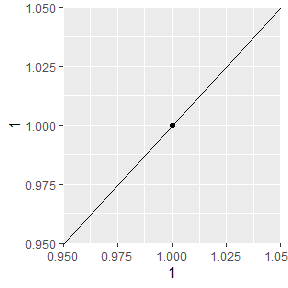Example of the Line of Identity

In the following sections, let us see how well esophageal and rectal temperature are in agreement after exercising in the heat for 1 hour at differing conditions.

## Rectal Temperature

Now, based on the typical thresholds (0.8 can be considered a “good” CCC), neither Trec as a raw value or a change score (Trec delta) is within acceptable degrees of agreement. As I will address later, this may not be an accurate and there are sometimes where there is a low CCC but the expected differences between conditions is acceptable (limits of agreement).

ccc_rec.post = cccrm::cccUst(dataset = df_temps,
ry = "trec_post",
rtime = "trial_condition",
rmet = "tod")

ccc_rec.post
#> CCC estimated by U-statistics:
#>         CCC   LL CI 95%   UL CI 95%      SE CCC
#> 0.218403578 0.007835121 0.410425602 0.104047391
ccc_rec.delta = cccrm::cccUst(dataset = df_temps,
ry = "trec_delta",
rtime = "trial_condition",
rmet = "tod")

ccc_rec.delta
#> CCC estimated by U-statistics:
#>        CCC  LL CI 95%  UL CI 95%     SE CCC
#> 0.66232800 0.49409601 0.78275101 0.07316927

Finally, we can visualize the concordance between the two different types of measurements and the respective time-of-day and conditions. From the plot we can see there is clear bias in the raw post exercise values (higher in the PM), but even when “correcting for baseline differences” by calculating the differences scores we can see a higher degree of disagreement between the two conditions.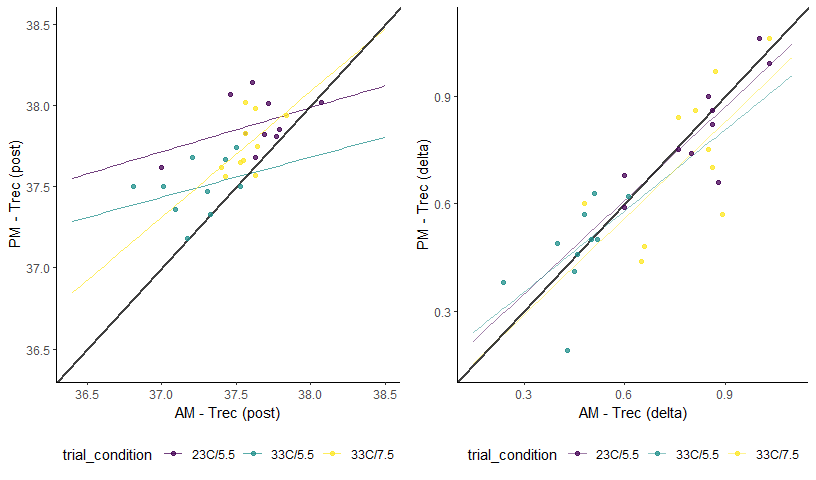Concordance Plots of Rectal Temperature

# Esophageal Temperature

We can replicate the same analyses for esophageal temperature. From the data and plots below we can see that the post exercise CCC is much improved compared to rectal temperature. However, there is no further improvement when looking at the delta (difference scores) for pre-to-post exercise.

ccc_eso.post = cccrm::cccUst(dataset = df_temps,
ry = "teso_post",
rtime = "trial_condition",
rmet = "tod")

ccc_eso.post
#> CCC estimated by U-statistics:
#>        CCC  LL CI 95%  UL CI 95%     SE CCC
#> 0.67333327 0.48765924 0.80073160 0.07915895
ccc_eso.delta = cccrm::cccUst(dataset = df_temps,
ry = "teso_delta",
rtime = "trial_condition",
rmet = "tod")

ccc_eso.delta
#> CCC estimated by U-statistics:
#>       CCC LL CI 95% UL CI 95%    SE CCC
#> 0.5654583 0.2663607 0.7652237 0.1276819
#> Warning: Removed 3 row(s) containing missing values (geom_path).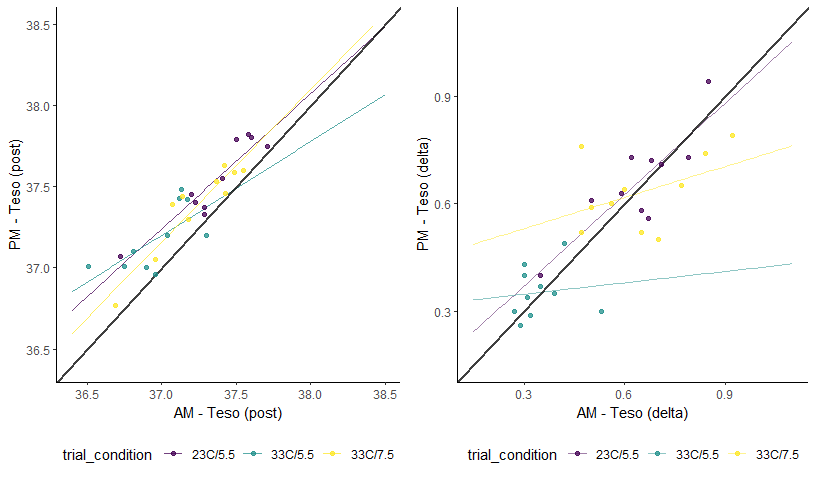Concordance Plots of Esophageal Temperature

# Limits of Agreement

In addition to the CCC we can use the loa_mixed function in order to calculate the “limits of agreement”. Typically the 95% Limits of Agreement are calculated which provide the difference between two measuring systems for 95% of future measurements pairs. In order to do that we will need the data in a “wide” format where each measurement (in this case AM and PM) are their own column and then we can calculate a column that is the difference score. Once we have the data in this “wide” format, we can then use the loa_mixed function to calculate the average difference (mean bias) and the variance (which determines the limits of agreement).

## Rectal Temperature

So we will calculate the limits of agreement using the loa_mixed function. We will need to identify the columns with the right information using the diff, condition, and id arguments. We then select the right data set using the data argument. Lastly, we specify the specifics of the conditions for how the limits are calculated. For this specific analysis I decided to calculate 95% limits of agreement with 95% confidence intervals, and I will use bias-corrected accelerated (bca) bootstrap confidence intervals.

rec.post_loa = SimplyAgree::loa_mixed(diff = "diff",
condition = "trial_condition",
id = "id",
data = df_rec.post,
conf.level = .95,
agree.level = .95,
replicates = 199,
type = "bca")

When we create a table of the results we can see that CCC and limits of agreement (LoA), at least for Trec post exercise, are providing the same conclusion (poor agreement).

knitr::kable(rec.post_loa$loa, caption = "LoA: Trec Post Exercise") LoA: Trec Post Exercise estimate lower.ci upper.ci Mean Bias 0.2300000 0.1727896 0.3391757 Lower LoA -0.1958766 -0.2948109 -0.1089945 Upper LoA 0.6558766 0.5243691 0.8450319 Furthermore, we can visualize the results with a typical Bland-Altman plot of the LoA. plot(rec.post_loa)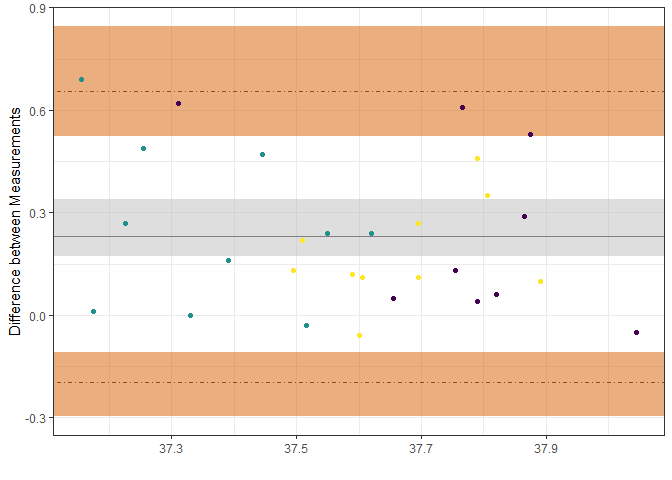Limits of Agreement for Trec Post Exercise Now, when we look at the Delta values for Trec we find that there is much closer agreement (maybe even acceptable agreement) when we look at LoA. However, we cannot say that the average difference would be less than 0.25 which may not be acceptable for some researchers. knitr::kable(rec.delta_loa$loa,
caption = "LoA: Delta Trec")
LoA: Delta Trec
estimate lower.ci upper.ci
Mean Bias -0.0210000 -0.0571838 0.0243662
Lower LoA -0.2552956 -0.3613519 -0.1805686
Upper LoA 0.2132956 0.1670232 0.2932911
plot(rec.delta_loa)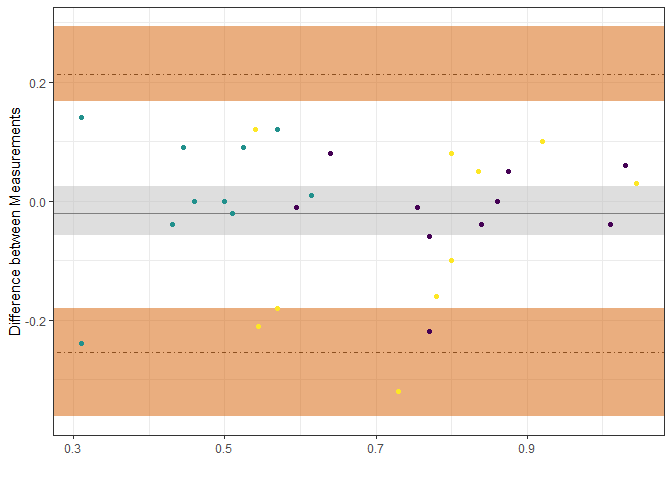Limits of Agreement for Delta Trec

## Esophageal Temperature

We can repeat the process for esophageal temperature. Overall, the results are fairly similar, and while there is better agreement on the delta (change scores), it is still fairly difficult to determine that there is “good” agreement between the AM and PM measurements.

eso.post_loa = SimplyAgree::loa_mixed(diff = "diff",
condition = "trial_condition",
id = "id",
data = df_eso.post,
conf.level = .95,
agree.level = .95,
replicates = 199,
type = "bca")
knitr::kable(eso.post_loa$loa, caption = "LoA: Teso Post Exercise") LoA: Teso Post Exercise estimate lower.ci upper.ci Mean Bias 0.1793333 0.1341438 0.2243988 Lower LoA -0.0795336 -0.1441305 0.0308588 Upper LoA 0.4382003 0.3465289 0.5292906 plot(eso.post_loa)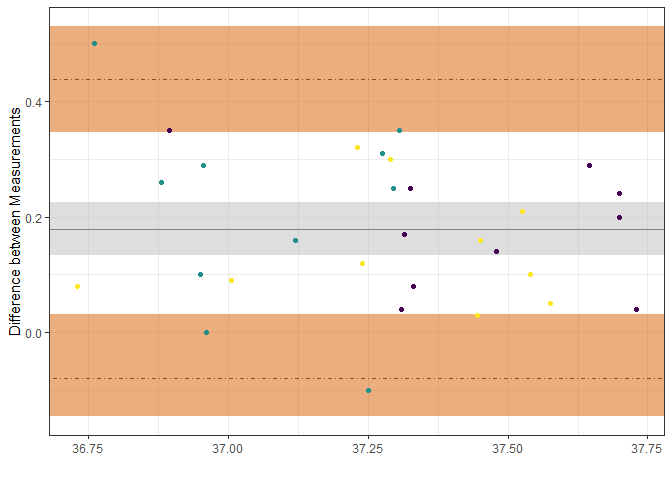Limits of Agreement for Teso Post Exercise knitr::kable(eso.delta_loa$loa,
caption = "LoA: Delta Teso")
LoA: Delta Teso
estimate lower.ci upper.ci
Mean Bias 0.0026667 -0.0324062 0.0370979
Lower LoA -0.2165808 -0.3011728 -0.1585443
Upper LoA 0.2219141 0.1515147 0.3399762
plot(eso.delta_loa)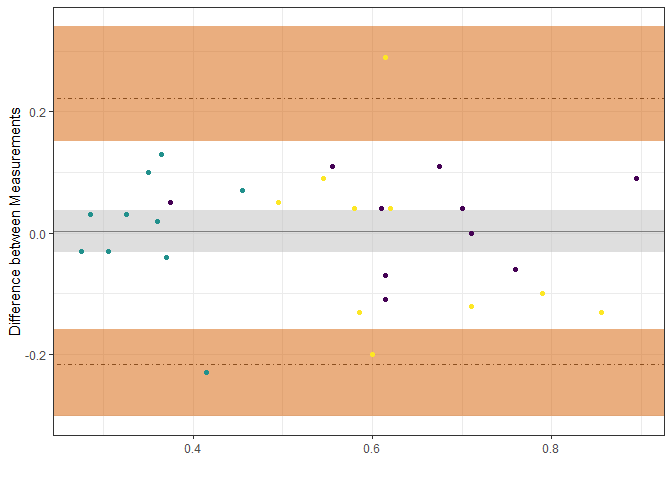Limits of Agreement for Delta Teso